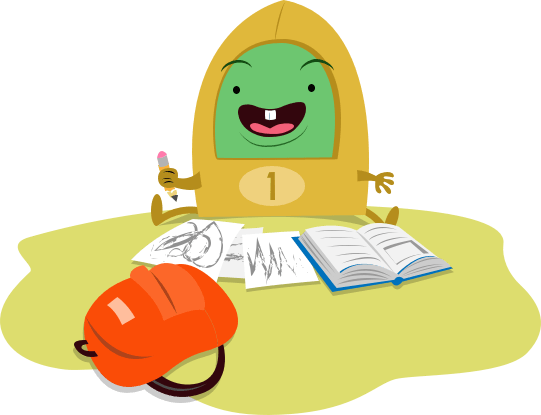# This Week in Eighth Grade

Week 12## Equations and Language

Learners practice solving one-variable, multi-step equations in two targeted worksheets. Then, they'll practice extended reading comprehension and explore the author's use of language and literary devices.
Students practice solving one-variable, multi-step equations in two targeted worksheets. Then, they'll practice extended reading comprehension and explore the author's use of language and literary devices.
Solving Multi-Step Equations: Part 1
Worksheet
Solving Multi-Step Equations: Part 1
Practice solving one-variable, multi-step equations with this eighth-grade algebra worksheet!
Math
Worksheet
Solving Multi-Step Equations: Part 2
Worksheet
Solving Multi-Step Equations: Part 2
Give students extra practice solving multi-step equations with this eighth-grade algebra worksheet.
Math
Worksheet
Worksheet
Students read a two-page adaptation of "The Gift of the Magi" and dig deep into the figurative language and literary devices of this classic story!
Worksheet
Literary Response Prompt: Language
Worksheet
Literary Response Prompt: Language
Students will choose one of two writing prompts focused on analyzing the author’s use of language and literary devices in this literary response worksheet.
WorksheetSend home these fun free resources to help reinforce the skills you're teaching in the classroom.